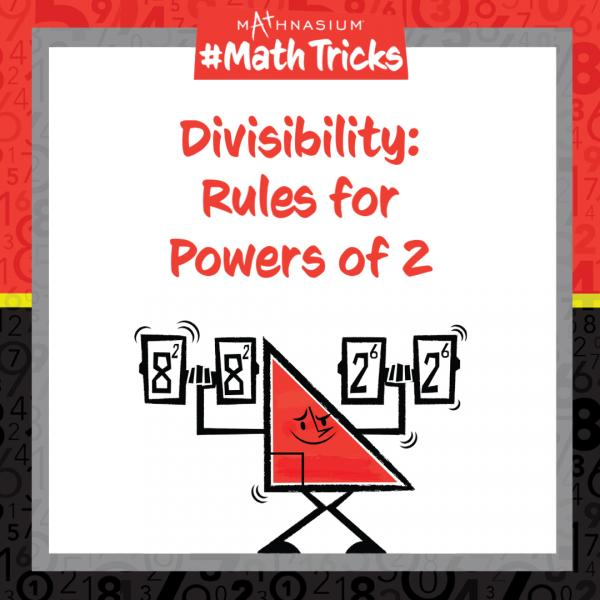# Mathnasium #MathTricks: Divisibility (Powers of 2)

By Mathnasium | Added Nov 16, 2022Welcome to Mathnasium’s Math Tricks, series. Today we are determining whether a number can be divided by a power of 2.

Recall that a number is a power of 2 if it can be expressed in the form 2n. For example, 64 is a power of 2 since it can be expressed as 26: 64 = 2 x 2 x 2 x 2 x 2 x 2.

A number is divisible by a power of 2 if the last “power”-amount of digits can be divided by the power of 2.

Follow the example below to determine whether the number is divisible by the power of 2.

##### Example: Is 41,328 divisible by 16?

Step 1: Determine the power of 2.

16 = 24

Step 2: Check whether the last “power”-amount of digits can be divided by the power of 2.

Since 16 is the fourth power of 2, we check whether the last four digits of 41,328 can be divided by 16.

1,328 is divisible by 16 since it is divisible by 2, four times:

1,328 ÷ 2 = 664
664 ÷ 2 = 332
332 ÷ 2 = 166
166 ÷ 2 = 83